1.800.221.5175
Core Program
Full Access
Core Program
Full Access
High-Impact Tutoring
Core Program
Full Access
National Program
Full Access
New York
Critical Thinking for Active Math Minds
Preparing for Standards Based Assessments
Vocabulary Workshop, Tools for Comprehension Grades 1–5
Print Program
Interactive Edition
Print Program
Interactive Edition
Vocabulary Workshop, Tools for Excellence Grades 6–12+
Print Program
Interactive Edition
Vocabulary for Success
Progress English Language Arts Grades K–8
National Program
Full Access
High-Impact Tutoring
New York
Grammar Workshop, Tools for Writing
Grammar for Writing
Writing a Research Paper
Writing Workshop
Print Program
Interactive Practice Bundle
Fluency Booster Practice Book
Interactive Assessments
High-Impact Tutoring
Building Reading Success with Wiley Blevins
Print Program
Interactive Edition
Professional Learning

A K–8 resource to support deep comprehension of math skills and conceptsTopics:

#### March 1, 2017 k-2-math-practices, 6-8-math-practices, mp-reasoning, 3-5-math-practices

I have been seeing the usefulness of teaching fact families at the middle school level. As a result, I have been thinking about how students look for and express regularity in repeated reasoning (Mathematical Practice 8)  in a new way.

## MAKING CONNECTIONS THROUGH REPEATED REASONING

The repeated reasoning that results from fact families is about inverse operations, addition and subtraction, multiplication and division. These inverse relationships continue to be repeated as students expand the types of numbers they use in their reasoning.

When this kind of reasoning can be repeated across the grade levels, it strengthens students’ thinking about these related operations and helps give them insight into the different kinds of numbers they will encounter later in middle school: fractions, rational numbers, integers, and scientific notation.

The ability to look for and express regularity in repeated reasoning through the use of fact families helps students to see the connections between what they learned in the past and what they are currently learning.

## WHAT I MEAN BY "FACT FAMILIES"

Before going on, I want to be clear about what I mean by “fact families.” I reinforce with my students that if they can write one number fact, they should automatically and quickly write three more related facts. Here are some examples:

2 x 3 = 6          →         3 x 2 = 6          →         6 ÷ 2 = 3                      →         6 ÷ 3 = 2

OR

3 + 5 = 8          →         5 + 3 = 8          →         8 - 5 = 3           →         8 - 3 = 5

## FACT FAMILIES ACROSS THE GRADE LEVELS

This understanding of fact families for addition and subtraction is in the Common Core Operations and Algebraic Thinking Standards for Grade 1 and the Grade 2 Number and Operations Standards.

The multiplication fact families are first noted in the Grade 3 Operations and Algebraic Thinking Standards.

Students can first look for and express regularity in repeated reasoning by understanding that the addition/subtraction relationship learned in Grades 1 and 2 also holds true for developing understanding of the relationship between multiplication and division.

Although the idea of fact families are often left behind after Grade 4, students can look for express regularity in repeated reasoning by continuing to write fact families as they explore some of the more difficult concepts in the middle school curriculum, such as dividing by fractions, subtracting negative numbers, and finding growth factors.

Here are some examples of how students' knowledge of fact families for addition/subtraction and multiplication/division learned in Grades 1–3 can be used in Grades 4–8 as repeated reasoning in order to help students understand other difficult mathematical concepts:

Grades 1 and 2: Students learn fact families for addition and subtract for the first time

4 + 5 = 9
5 + 4 = 9
9 - 5 = 4
9 - 4 = 5

Grade 3: Students learn fact families for multiplication and division

4 x 5 = 20
5 x 4 = 20
20 ÷ 4 = 5
20 ÷ 5 = 4

Grade 4: Students solve multiplicative comparison problems using division

5 x ? = 35
? x 5 = 35
35 ÷ ? = 5
35 ÷ 5 = ?

Grade 5: Students learn about multiplying/dividing powers of ten with decimal numbers

4.35 x 10 = 43.5
10 x 4.35 = 43.5
43.5 ÷ 10 = 4.35
43.5 ÷ 4.35 = 10

6 x (1/2) = 3
(1/2) x 6 = 3
3 ÷ (1/2) = 6
3 ÷ 6 = 1/2

(-2) + (-7) = -9
(-7) + (-2) = -9
(-9) – (-2) = -7
(-9) – (-7) = -2

Grade 8: Students solve multiplicative comparisons problems with scientific notation

(3 x 104) x ? = 1.2 x 10-2
? x (3 x 104) = 1.2 x 10-2
(1.2 x 10-2) ÷ ? = (3 x 104)
(1.2 x 10-2) ÷ (3 x 104) = ?

## MATH COACHES AND COORDINATORS

If you are a coach or a curriculum coordinator, you have a real opportunity to highlight how this previously-learned idea can be brought to the higher grades. You can use the download from this post to show upper grade teachers how to apply this form of repeated reasoning to their grade levels.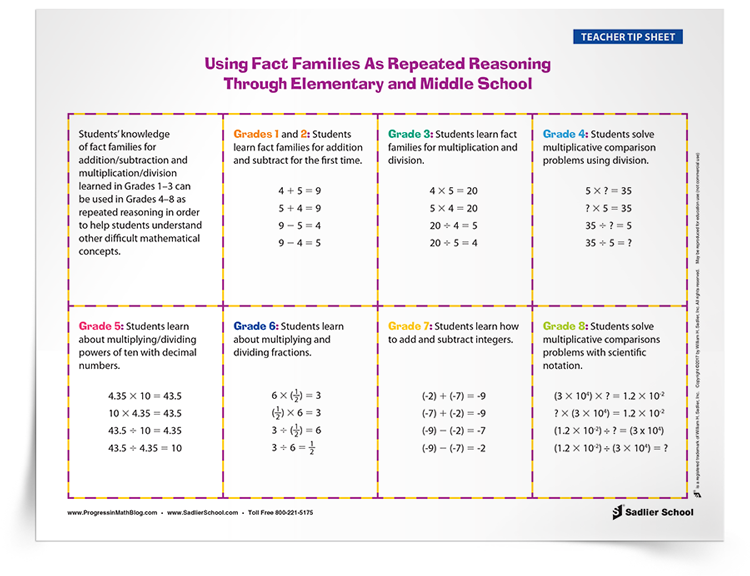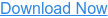## IN SUMMARY

When students look for and express regularity in repeated reasoning through the use of fact families, it helps students to see the connections between what they learned in the past and what they are currently learning.

Download a tip sheet to see examples of how I use fact families to help students look for and express regularity in repeated reasoning in order to help learn these concepts.Mathematical Practice 1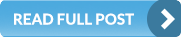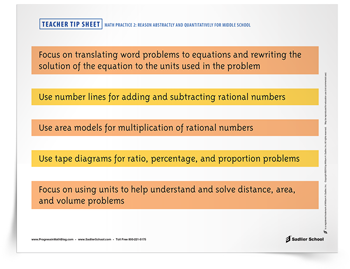Mathematical Practice 2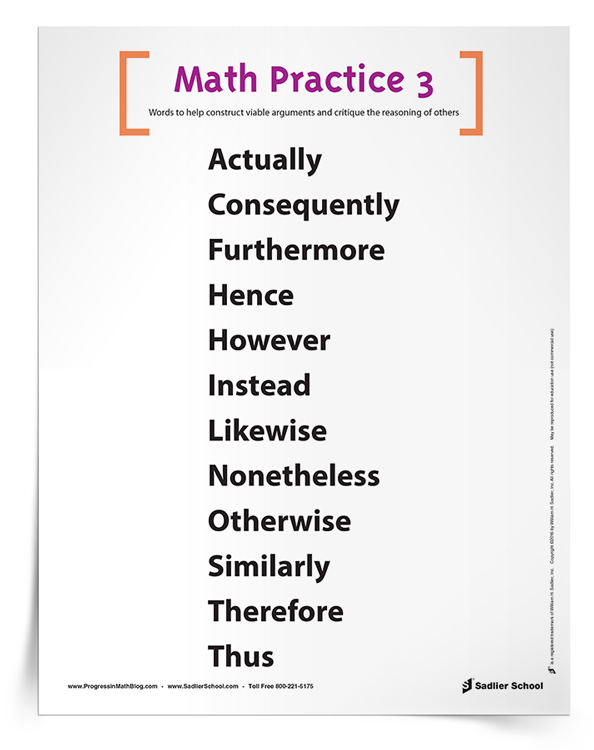Mathematical Practice 3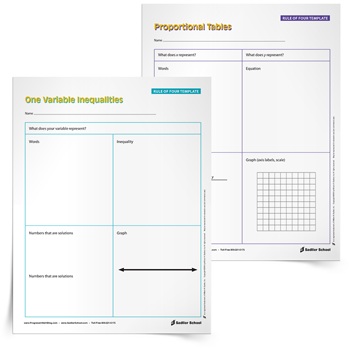Mathematical Practice 4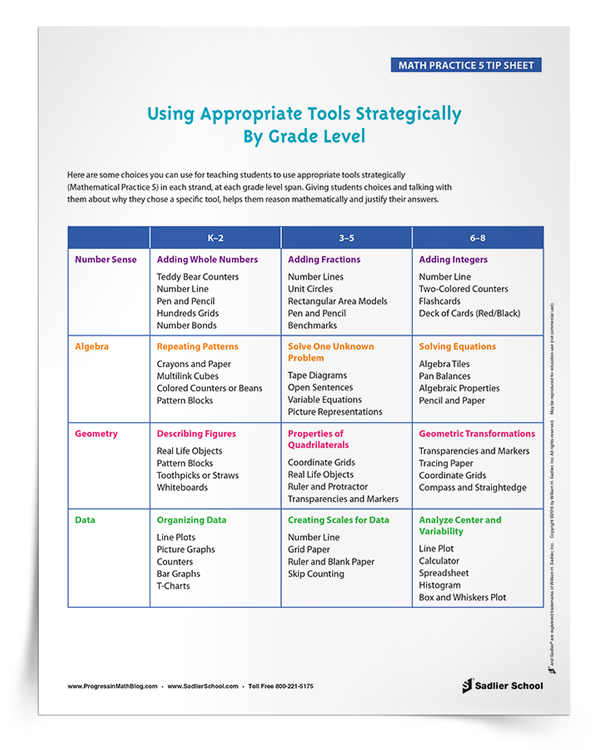Mathematical Practice 5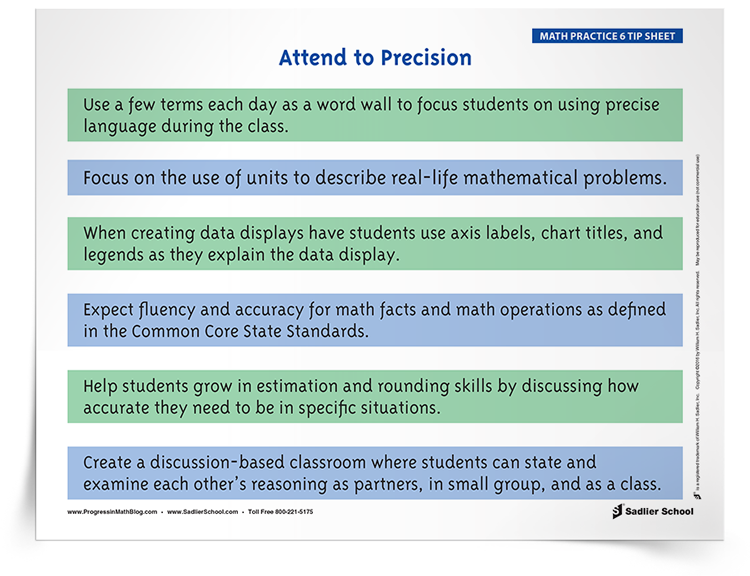Mathematical Practice 6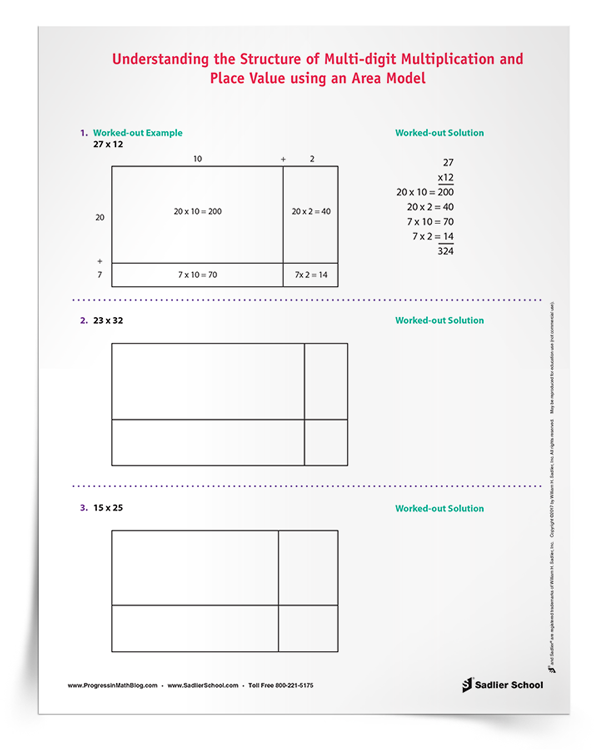Mathematical Practice 7